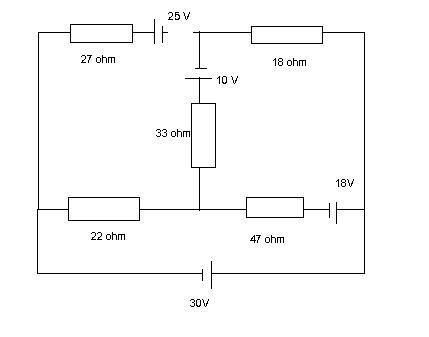# Resistor value

resistor value ....

## Homework Statementsee the attached diagram

## Homework Equations

What value of resistor in the place of the 33 ohm resistor would draw maximum power from the rest of the circuit and what power would it draw?

## The Attempt at a Solution

I can figure out the currents flowing in different branches of the circuit using maxwell mesh network analysis. But I did not have a clue of how to solve the problem. I know what power dissipation is and how to work it out, but the given question is giving me headaches.

Last edited:

MATLABdude

Nobody can see your attachment yet, pending administrator approval. You could always host it somewhere else, and then link to it.

MATLABdude

Ah, your attachment has been approved!

I don't know if you're still looking for help or not, but I'll guide you a bit:

1) Under what load conditions does maximum power transfer occur?

2) How would you black box the rest of the circuit, making it a block that you could attach this resistor to?

EDIT: For future reference, you may want to host your picture elsewhere and post a link, instead of waiting for moderator approval.

MATLABdude first thanks for the reply,

I know that the maximum power transfer occurs when the load resistance is equal to that of the voltage source internal resistance.

Are you saying that looking from the 33ohm resistor point of view and calculating the total resistance of all the resistor(not including 33ohm resistor) and hence that will be the valueof the resistor that the 33 ohm resistor should be?
Do you mean to calculate the total resistance(obviously ommiting 33 ohm resistor) by replacing the batteries with short circuits. It sounds like thevenin problem or is it?

MATLABdude